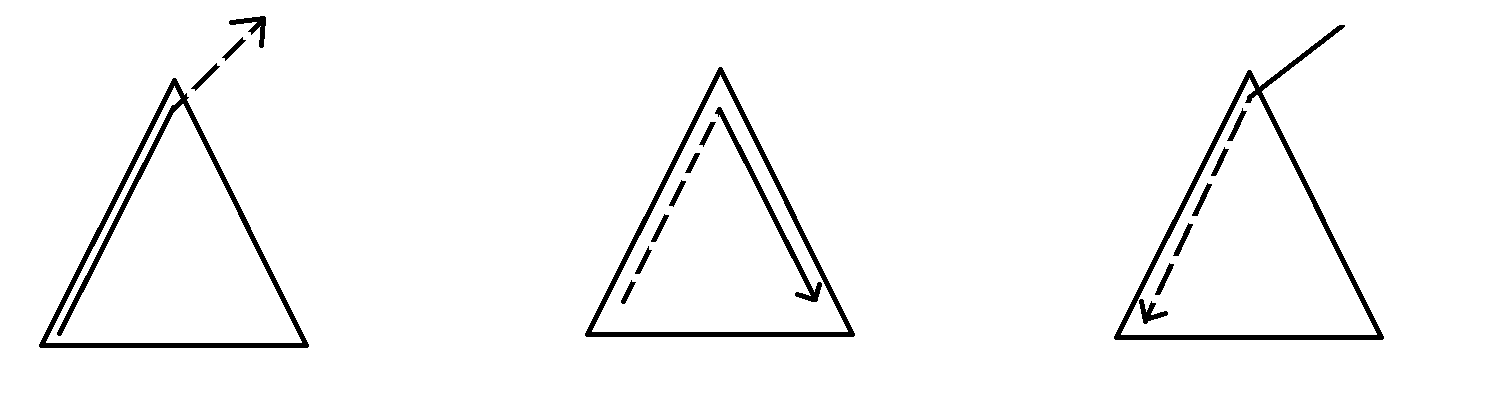# categories/刷题记录/共653篇文章zc
2019-12-21 19:47:00zc
2019-12-21 19:47:00

(tips:getline,ssstream)zc
2019-12-21 19:47:00

1.如果全是0

2.没有0

3.没有负数

4.没有正数zc
2019-12-21 19:47:001. x子树内\rightarrow x \rightarrow x子树外(包括x)

2. x子树内(包括x)\rightarrow x \rightarrow x子树内

3. x子树外\rightarrow x \rightarrow x子树内

1. ans += V_x \times (f_x-g_x) \times (n-siz_x+1)(可以在x的子树中随机选一个往子树外面走(可以指走到x))

2. ans += V_x \times (g_{to}-f_{to}) \times (siz_x-siz_{to}-1)

ans+= V_x \times (siz_x-1)(可以从x直接向下走)

3. 我们需要记录x子树外与x的距离为偶数和与x的距离为奇数的点的个数,分别记作uf_x,ug_x

我们可以通过bfs从上到下来处理,那么

uf_x=ug_{fa_x}+g_{fa_x}-f_x

ug_x=uf_{fa_x}+f_{fa_x}-g_x

ans += V_x \times (uf_x-ug_x) \times siz_xzc
2019-12-21 19:47:00zc
2019-12-21 19:47:00

f[i][j]表示在i的子树中与i距离为j的点数

g[i][j]表示以i为根的子树中,点对(x,y)满足x,ylca(x,y)距离都是d,并且lca(x,y)i的距离为d-j的点对数

g[i][j] = \sum g[to][j+1]

ans = \sum_{i=1}^n (g[i]+g[i][j] \times f[to][j-1])

n \le 100000zc
2019-12-21 19:47:00

1. b_i = a_i + 1

一条链

直接二分最小值,然后判断即可

2. m=1

直接找树的直径

3. a_i = 1

记录所有边权,设边权为w,然后排序

w_1 + w_{2m} , w_2 + w_{2m-1}, ...的最小值

4. 分支不超过3(基本上就是正解了)

dfs(x,f,w)求出x的子树连接x长度不超过w的最长路径

stl,还是太弱了Q\omega Qzc
2019-12-21 19:47:00

f[i][sta]表示以第i只奶牛结尾,状态为sta的情况下有多少种

f[i][sta] = \sum f[i-1][sta']zc
2019-12-21 19:47:00

H:minH , V:minV

A \times (h-H) + B \times (v-V) \le C

A \times h + B \times v -C \le A \times H + B \times V

s = Ah + Bv - C,按s排序,确保i可以取j就可以取(j<i)zc
2019-12-21 19:47:00

1. 都是最小深度

2. 都是最大深度

3. 两种都有

Search
search